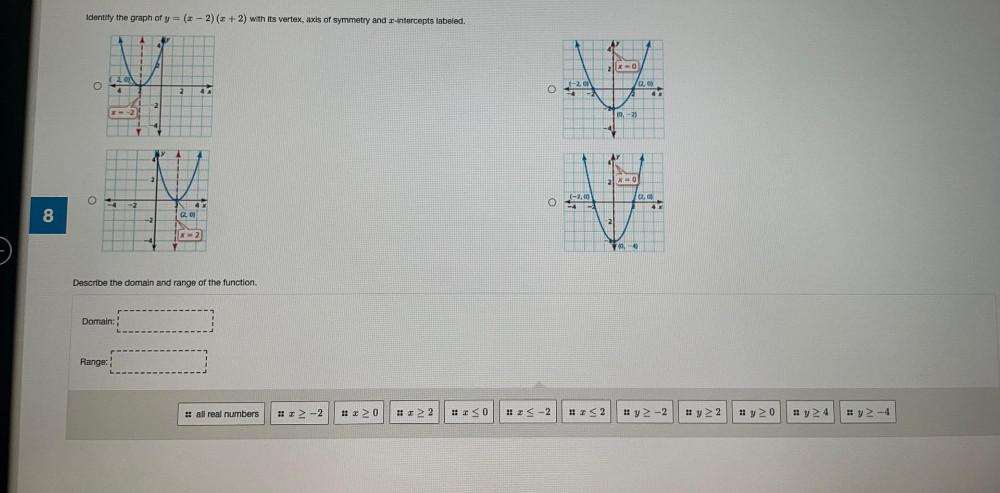Question:

# Identify the graph of y=(x-2)(x + 2) with its vertex, axis of symmetry and z-intercepts labeled. 2x ( 201 O (-20) 2 4 O 2,0) 22Identify the graph of y=(x-2)(x + 2) with its vertex, axis of symmetry and z-intercepts labeled. 2x ( 201 O (-20) 2 4 O 2,0) 22 4 -21 2 X-2 10,-2) 21 x 0 (-2,0) (2.0) O -2 O 8 2.0) -2 2 [x = 2 -4 70-4 Describe the domain and range of the function. Domain: Range: :: all real numbers !! 3-2 :: 3 > 0 :: I> 2 :: 3 < 0 :: 3 < -2 !! <2 y> -2 y > 2 Ayo Iy24 y-4